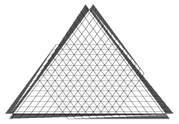## neal young / Karger04Rounding

•The multiway-cut problem is, given a weighted graph and $$k > 2$$ terminal nodes, to find a minimum-weight set of edges whose removal separates all the terminals. The problem is NP-hard, and even NP-hard to approximate within $$1+\delta$$ for some small $$\delta > 0$$.

This paper gives a 12/11-approximation algorithm for $$k=3$$ and a 1.3438-approximation algorithm in general. Working on this result was particularly fun because the problem of designing the algorithm was itself formulated as an optimization problem and approximately solved by computer. These computations guided the final (non-computational) solutions.

The paper also gives a proof that for a general class of geometric embedding'' relaxations, there are always randomized rounding schemes that match the integrality gap. (Another example of such a geometric-embedding relaxation is the semidefinite-programming relaxation of max-cut by Goemans and Williamson .)

The geometric relaxation is due to Calinescu, Karloff, and Rabani . After our paper, the approximation ratio for general $$k$$ was subsequently improved to 1.3238 in .

Journal version of .